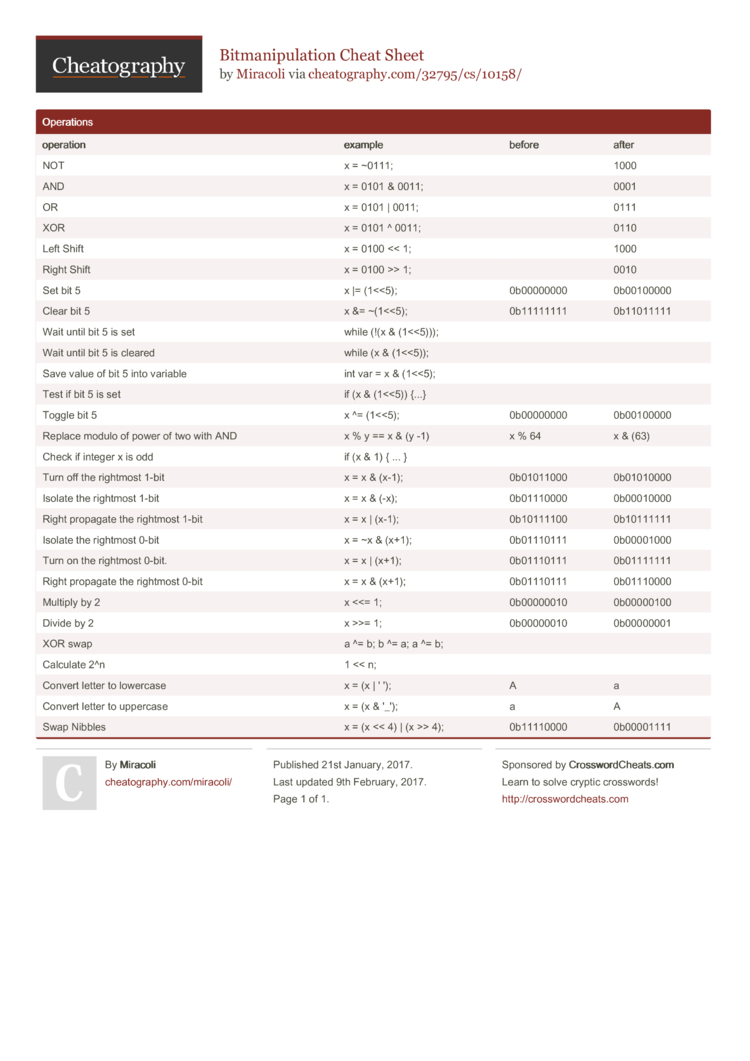# Bitmanipulation Cheat Sheet by Miracoli

### Operations

 oper­ation exam­ple before after NOT x = ~0111; 1000 AND x = 0101 & 0011; 0001 OR x = 0101 | 0011; 0111 XOR x = 0101 ^ 0011; 0110 Left Shift x = 0100 << 1; 1000 Right Shift x = 0100 >> 1; 0010 Set bit 5 x |= (1<­<5); 0b00000000 0b00100000 Clear bit 5 x &= ~(1<<5); 0b11111111 0b11011111 Wait until bit 5 is set while (­!(x & (1<­­<5))); Wait until bit 5 is cleared while (x & (1<­­<5)); Save value of bit 5 into variable int var = x & (1<­­<5); Test if bit 5 is set if (x & (1<­­<5)) {...} Toggle bit 5 x ^= (1<­­<5); 0b00000000 0b00100000 Replace modulo of power of two with AND x % y == x & (y -1) x % 64 x & (63) Check if integer x is odd if (x & 1) { ... } Turn off the rightmost 1-bit x = x & (x-1); 0b01011000 0b01010000 Isolate the rightmost 1-bit x = x & (-x); 0b01110000 0b00010000 Right propagate the rightmost 1-bit x = x | (x-1); 0b10111100 0b10111111 Isolate the rightmost 0-bit x = ~x & (x+1); 0b01110111 0b00001000 Turn on the rightmost 0-bit. x = x | (x+1); 0b01110111 0b01111111 Right propagate the rightmost 0-bit x = x & (x+1); 0b01110111 0b01110000 Multiply by 2 x <<= 1; 0b00000010 0b00000100 Divide by 2 x >>= 1; 0b00000010 0b00000001 XOR swap a ^= b; b ^= a; a ^= b; Calculate 2^n 1 << n; Convert letter to lowercase x = (x | ' '); A a Convert letter to uppercase x = (x & '_'); a A Swap Nibbles x = (x << 4) | (x >> 4); 0b11110000 0b000011111 Page
//media.cheatography.com/storage/thumb/miracoli_bitmanipulation.750.jpg

PDF (recommended)

I really like it! *.*
I was searching for something like this :D
Thank's